×

Search anything:

# K queues in an array

#### queue AlgorithmsGet this book -> Problems on Array: For Interviews and Competitive Programming

In this article, we have explained 2 approaches to implement K queues in a single array. The challenge is that the space of the array should be utilized optimally by the K queues.

### Table of content

Method A
A1. A simple approach
A2. Algorithm
A3. Time & Space complexity
A4. A way of implementation

Method B
B1. An efficient approach
B2. Algorithm
B3. Time & Space complexity
B4. A way of implementation

Pre-requisites:

### Method A

#### A1. A simple approach

As we could see in this article implementing 2 queues in an array required implementing 2 push and pop methods for each queue in the array.
How about doing this hard coding for k queues ? Doesn't sound too good, right ?
A logical approach to solve this is to use another parameter k representing the queue that needs to be modified and sending it to the push and pop methods.
But that will not be sufficient since we do not know exactly the heads of the k queues. So, we must calculate them. Since we cannot have a hard coded variables the only way is to use an array that will store the indexes for the queues.

The simplest way to do that is to see if there are remained elements that don't belong to any queue, and that because an array might have even or odd numbers of elements. The number of them can be simple to find by using the mod function and then increase the index of the first rest number of queues by 1.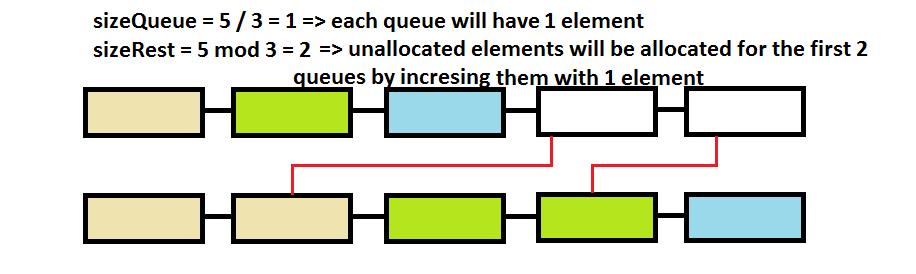For example:

• having an array of 6 elements and 3 queues will mean that each queue will have 2 elements with the head indexes for the queues [2,4,6]
• having an array of 5 elements and 3 queues will mean that not all the queues will be equals. We will have in this case 2 elements unallocated, so the last queue will have with 1 element less than the first ones having the head indexes for the queues [2,4,5]

The downsize of this approach is that it is space inefficient, meaning the size of the queues are fixed and if we want to insert one element in a full queue we cannot do that even though there are free spaces in other queues.

#### A2. Algorithm

The algorithm for push and pop methods will be the same as in the mentioned article the only difference consisted in calculating the indexes for each queue.
For that we are going to use 2 arrays:

• one for storing the heads of each queue
• one for storing the movement of elements inside each queue

Pseudo code for calculating the indexes of the k queues

``````    if k > 0 and sizeArray >= k

sizeQueue = sizeArray / k;
sizeRest = sizeArray mod k;

array idxQk[k] that will store the moved index of the k queue
array idxQh[k] that will store the head index of the k queue

i = 0;
loop
if i < sizeRest
idxQk[i] <- sizeQueue +1;
idxQh[i] <- idxQk[i];
i <- i+1
end loop

i = sizeRest;
loop
if i < k
idxQk[i] <- sizeQueue ;
idxQh[i] <- idxQk[i];
i <- i+1;
end loop
``````

#### A3. Time & Space complexity

For calculating indexes for k queues the time and space will be O(n).
For push method the time will be O(1) since we are doing one operation.
For pop method the time will be O(n) since we are moving elements.

#### A4. A way of implementation

``````#include <iostream>

using namespace std;

class kQueuesArray
{
private:
int *v, sizeV, sizeQ, sizeR, *idxQk, *idxQh;
public:
kQueuesArray(int size, int k)
{int i;

if (size > 0)
{
v = new int[size];
sizeV = size;
if (k > 0 && size >= k)

sizeQ = sizeV / k;
sizeR = sizeV % k;

idxQk = new int [k]; //store the moved index of the k queue
idxQh = new int [k]; //store the head index of the k queue

for (i = 0; i < sizeR; i++)
{
idxQk[i] = (i-1 >= 0 ? idxQk[i-1] : 0 ) + sizeQ +1;
idxQh[i] = idxQk[i];
}

for (i = sizeR ; i < k; i++)
{
idxQk[i] = (i-1 >= 0 ? idxQk[i-1] : 0 ) + sizeQ ;
idxQh[i] = idxQk[i];
}
/*
some languages start indexing arrays with 0 so
we need to adjust calculated indexes by decreasing them with 1
*/
for (i = 0; i < k; i++)
{
idxQk[i]--;
idxQh[i] = idxQk[i];
}
}
}
void pushQk(int elem, int Qk)
{
if (v[idxQk[Qk-1]]==0 && idxQk[Qk-1] >= 0 )
v[idxQk[Qk-1]--] = elem;
//else Queue k is full
}
int popQk(int Qk)
{
int temp;
if ( v[idxQh[Qk-1]] )
{
temp = v[idxQh[Qk-1]] ;

if(idxQk[Qk-1] < idxQh[Qk-1]-1 )
for (int i=idxQh[Qk-1]; i-1 > idxQk[Qk-1] ;i--) //move all the elements to the right
{
v[i] = v[i-1];
v[i-1] = 0;
}
else
v[idxQh[Qk-1]] = 0;
idxQk[Qk-1]++;
}
//else Queue k is empty
return temp;
}

void coutV()
{
for (int i=0;i<sizeV;i++)
cout<<"v["<<i<<"]="<<v[i]<<" ";
cout<<endl;
}
};

int main()
{
kQueuesArray a(5,3);
a.pushQk(11,1);
a.pushQk(12,1);
a.pushQk(13,1);
a.pushQk(21,2);
a.pushQk(22,2);
a.pushQk(31,3);
a.coutV();

cout<<a.popQk(2)<<endl;
a.coutV();

a.pushQk(23,2);
a.coutV();

cout<<a.popQk(3)<<endl;
a.coutV();

a.pushQk(32,3);
a.coutV();

return 0;
}

``````

Step by step example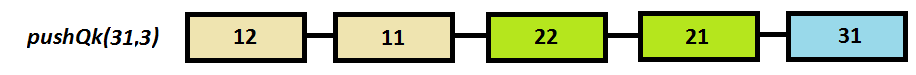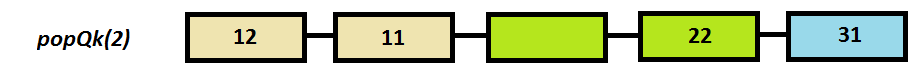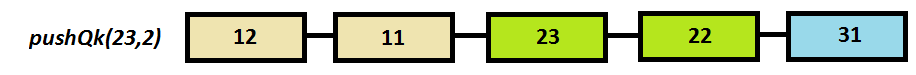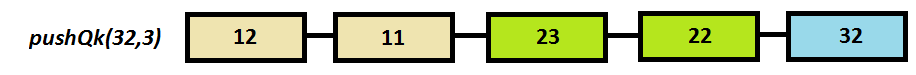### Method B

#### B1. An efficient approach

As we could see from the previous method, again, size of the k queues are fixed in size.
Can we find a way to store elements in an array in such a way to store them dynamically?
As we could see in the 2-nd method from the mentioned article, an array has only 2 heads and we could implemented the efficient storing of elements only for 2 queues.
So, that approach will not work for k queues.
What if we will use the pointer arithmetic logic and store for each element in the array the next element from the k queue that points to it ?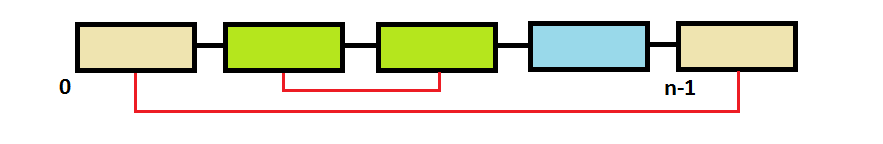As you could see in the image, the elements that belong to same queue are not store side by side as in previous methods.
The main logic of this approach is to store for each queue, the front, the rear and the next elements inside of it.

#### B2. Algorithm

Initialization

• front and rear arrays are initialized with -1 indicating the empty queues
• next array will be initialize with the next index in the array except for the last element which will be -1 indicating nothing to point to.
• empty_slot a variable which stores the index of next empty slot in the array, at first will be 0

push(elem, k)

``````    if empty_slot != -1
array[empty_slot] <- elem

last_empty_slot = empty_slot
last_front = front[k]

front[k] <- empty_slot

if rear[k] == -1
rear[k] <- empty_slot

empty_slot <- next[empty_slot];

next[last_empty_slot] = -1;

if (front[k] != fear[k])
next[last_front] = last_empty_slot;
else
queue k is full
``````

Since push is a forward implementation:

• front will store the current empty slot
• rear will store also the current empty slot if it was empty and nothing else if the queue already has a rear head
• next will store -1 if the element is the last head in the queue or it doesn't belong to any queue, else the index of the next element
• empty_slot will store the next available slot in the array

pop(k)

``````    if rear[k] != -1
array[rear[k]] <- nil

last_rear = rear[k]

if next[rear[k]] == -1
front[k] <- -1

rear[k] <- next[rear[k]]

empty_slot <- last_rear

idxNext[last_rear] <- -1

else
queue k is empty
``````

Since pop is a backward implementation:

• front will store -1 if the next rear head is empty else nothing to change
• rear will store the next rear head
• next will store -1 in the last rear element since it doesn't point to anything
• empty_slot will store the last rear head

#### B3. Time & Space complexity

For push method the time will be O(1) since we are doing one operation.
For pop method the time will be O(1) since we are doing one operation.

The space comlexity is O(n).

#### B4. A way of implementation

``````    #include <iostream>

using namespace std;

class kQueuesArray
{
private:
int *v, sizeV, sizeQ, *idxQFront, *idxQRear, *idxNext, empty_slot;
public:
kQueuesArray(int size, int k)
{
int i;
if (size > 0)
{
v = new int[size];

sizeV = size;

if (k > 0 && size >= k)
sizeQ = k;

idxQFront = new int [k]; //store the front index of the k queue
idxQRear = new int [k]; //store the rear index of the k queue
idxNext = new int[size];

for (i = 0; i < k; i++)
{
idxQFront[i] = idxQRear[i] = -1;
}

for (i = 0 ; i < size-1; i++)
{
idxNext[i] = i+1;
}
idxNext[sizeV-1] = -1; //mark the end of array
empty_slot = 0; //mark the next empty slot in the array
}
}
void pushQk(int elem, int Qk)
{
int last_front, last_empty_slot;

if(empty_slot != -1)
{
v[empty_slot] = elem,

last_empty_slot = empty_slot,
last_front = idxQFront[Qk-1],

idxQFront[Qk-1] = empty_slot,
idxQRear[Qk-1] = (idxQRear[Qk-1] == -1 ? empty_slot : idxQRear[Qk-1]),
empty_slot = idxNext[empty_slot];
idxNext[last_empty_slot] = -1;

if (idxQFront[Qk-1] != idxQRear[Qk-1])
idxNext[last_front] = last_empty_slot;
}
else cout<<"Queue is full"<<endl;

}
int popQk(int Qk)
{   int temp, last_rear;

if(idxQRear[Qk-1] != -1)
temp = v[idxQRear[Qk-1]],
v[idxQRear[Qk-1]] = 0,
last_rear = idxQRear[Qk-1],
idxQFront[Qk-1] = idxNext[idxQRear[Qk-1]] == -1 ? -1: idxQFront[Qk-1],
idxQRear[Qk-1] = idxNext[idxQRear[Qk-1]],
empty_slot = last_rear,
idxNext[last_rear] = -1;
else cout<<"Queue is empty"<<endl;

return temp;
}

void coutV()
{
for (int i=0;i<sizeV;i++)
cout<<"v["<<i<<"]="<<v[i]<<" ";
cout<<endl;
}
};

int main()
{
kQueuesArray a(5,3);
a.coutV();

a.pushQk(11,1);
a.pushQk(21,2);
a.pushQk(12,1);
a.pushQk(13,1);
a.pushQk(22,2);
a.pushQk(31,3);

a.coutV();

cout<<a.popQk(1)<<endl;
a.coutV();

a.pushQk(23,2);
a.coutV();

cout<<a.popQk(1)<<endl;
a.coutV();

a.pushQk(24,2);
a.coutV();

cout<<a.popQk(1)<<endl;
a.coutV();

a.pushQk(31,3);
a.coutV();

a.popQk(2);
a.coutV();

a.pushQk(32,3);
a.coutV();

return 0;
}
``````

Step by step example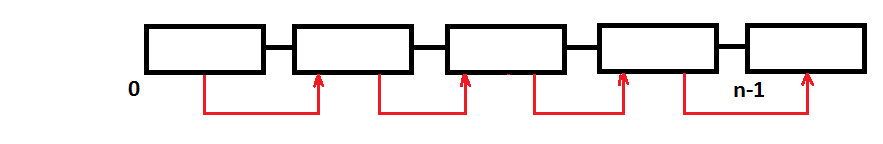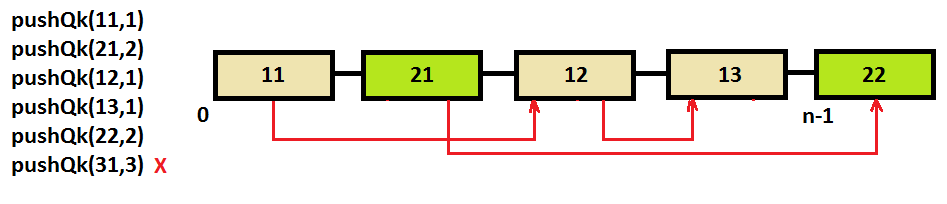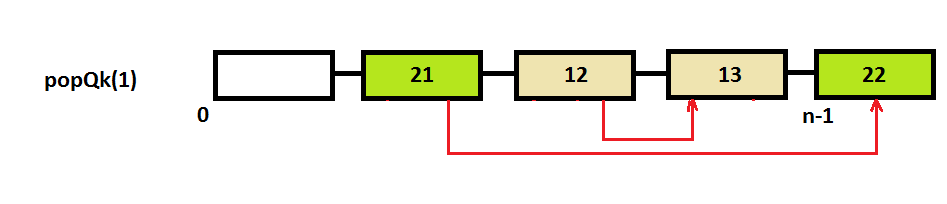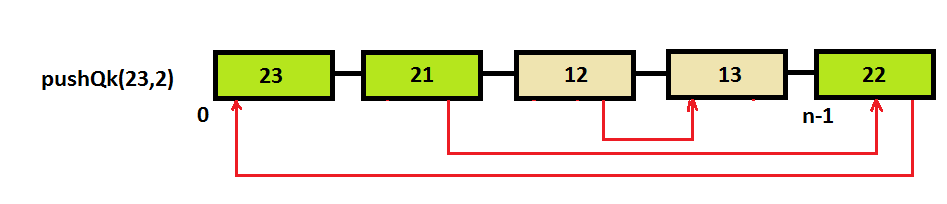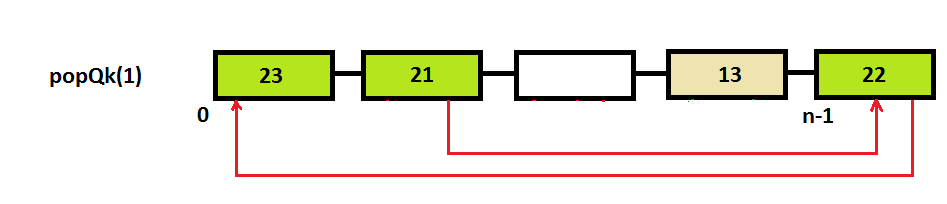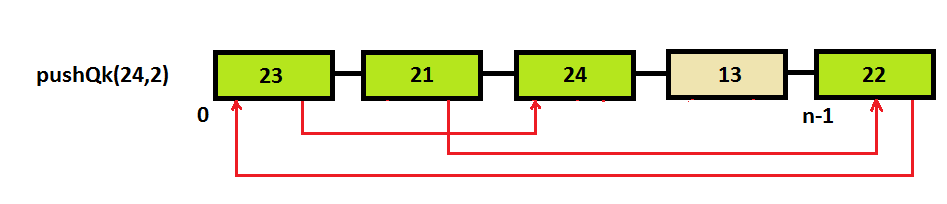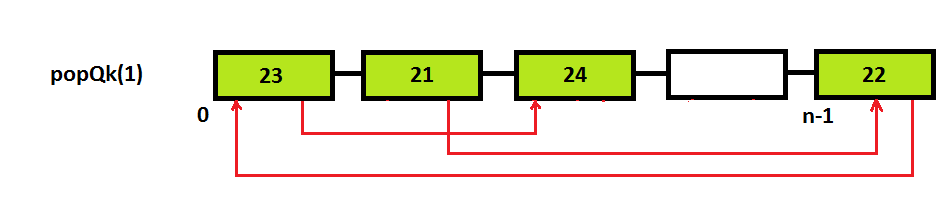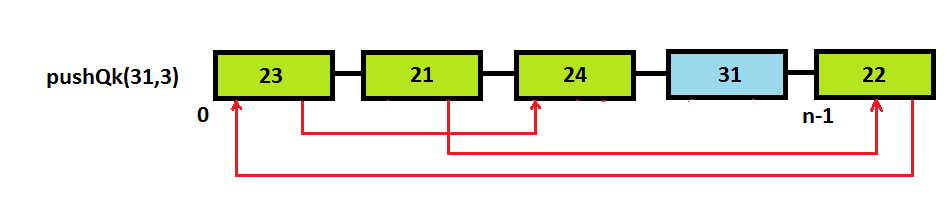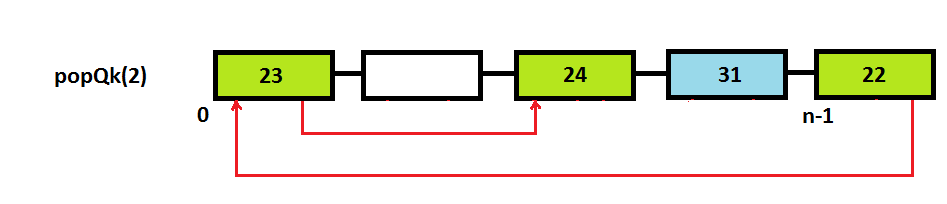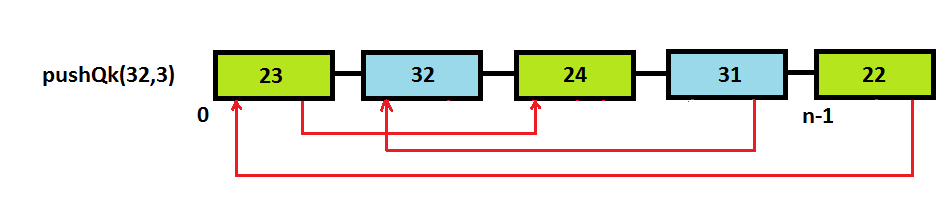With this article at OpenGenus, you must have the complete idea of how to implement K queues in an array.